Publication date: 04/12/2021

## Control Limits for P and NP Charts

The lower and upper control limits, LCL, and UCL, respectively, are computed using the following formulas:

P chart LCL =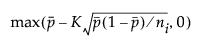P chart UCL =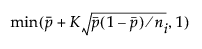NP chart LCL =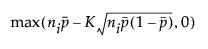NP chart UCL =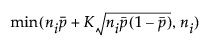where: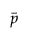is the average proportion of nonconforming items taken across subgroups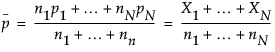ni is the number of items in the ith subgroup

K is the sigma multiplier and is set to 3 by default

Want more information? Have questions? Get answers in the JMP User Community (community.jmp.com).